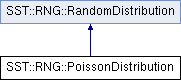SST  11.1.0 StructuralSimulationToolkit
SST::RNG::PoissonDistribution Class Reference

Creates an Poisson distribution for use within SST. More...

`#include "sst/core/rng/poisson.h"`

Inheritance diagram for SST::RNG::PoissonDistribution:## Public Member Functions

PoissonDistribution (const double mn)
Creates an Poisson distribution with a specific lambda. More...

PoissonDistribution (const double mn, SST::RNG::Random *baseDist)
Creates an Poisson distribution with a specific lambda and a base random number generator. More...

~PoissonDistribution ()
Destroys the Poisson distribution.

double getNextDouble ()
Gets the next (random) double value in the distribution. More...

double getLambda ()
Gets the lambda with which the distribution was created. More...Public Member Functions inherited from SST::RNG::RandomDistribution
virtual ~RandomDistribution ()
Destroys the distribution.

RandomDistribution ()
Creates the base (abstract) class of a distribution.

## Protected Attributes

const double lambda
Sets the lambda of the Poisson distribution.

SST::RNG::RandombaseDistrib
Sets the base random number generator for the distribution.

bool deleteDistrib
Controls whether the base distribution should be deleted when this class is destructed.

## Detailed Description

Creates an Poisson distribution for use within SST.

This distribution is the same across platforms and compilers.

## Constructor & Destructor Documentation

 PoissonDistribution::PoissonDistribution ( const double mn )
inline

Creates an Poisson distribution with a specific lambda.

Parameters
 mn The lambda of the Poisson distribution
 PoissonDistribution::PoissonDistribution ( const double mn, SST::RNG::Random * baseDist )
inline

Creates an Poisson distribution with a specific lambda and a base random number generator.

Parameters
 lambda The lambda of the Poisson distribution baseDist The base random number generator to take the distribution from.

## Member Function Documentation

 double PoissonDistribution::getLambda ( )
inline

Gets the lambda with which the distribution was created.

Returns
The lambda which the user created the distribution with
 double PoissonDistribution::getNextDouble ( )
inlinevirtual

Gets the next (random) double value in the distribution.

Returns
The next random double from the distribution

Implements SST::RNG::RandomDistribution.

The documentation for this class was generated from the following files:
• src/sst/core/rng/poisson.h
• src/sst/core/rng/poisson.cc